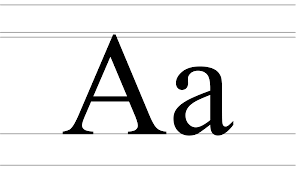# C Program Check A Character Is Upper Case Or Lower Case

C Program to Check Whether A Character is an Upper Case or Lower Case – In this distinct article, we will detail in on the several ways to check whether a character is an upper case or lower case.

Check out the blog to access the sample programs and suitable examples. The compiler has been added as well for you to execute the various programs.

The ways in which the character is checked for being an upper case or lower case are as follows:

• Using Standard Method
• Using User-Defined Method
• Using Pointers

As you all know, in the English language, there are a couple of cases of the alphabets. They are known as Upper Case and Lower Case. This is how both of those look like:As you can see, the left character is the Upper Case A and the right character is the Lower Case a. This specific article deals with the C programs to distinguish between both of these. The various ways are as follows:

## Using Standard Method

Output:

### Using User-Defined Function

1) Read the entered alphabet and store in the variable ch, using scanf function.

2)Pass the alphabet to the user-defined function lucase.

3)lucase function prints “uppercase” if entered alphabet ASCII value is >=65 and <=90,

prints “Lowercase” if entered alphabet ASCII value is >=97 and <=122,otherwise it prints invalid input.

Output:

#### Using Pointers

1)Pass the address of entered alphabet to the function lucase as lucase(&ch).

2)Then the pointer variable *ch  of lucase(char *ch)contains the ASCII value of the entered alphabet.

3)If the ASCII value of ch >=65 and <=90 then it prints “upper case”, if ASCII value of ch>=97 and <=122 then it prints “lower case” otherwise it prints “invalid input”.

Output: